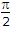# Electronics and Communication Engineering - Power Electronics

Exercise : Power Electronics - Section 5
1.
Multiphase choppers are used in
low power circuits
high power circuit
both low and high power circuits
medium power circuits
Explanation:
No answer description is available. Let's discuss.

2.
In a single phase converter using a centre tapped transformer and with a highly inductive load, the firing angles is a. Each thyristor conducts for a period
p
a
p - aExplanation:
No answer description is available. Let's discuss.

3.
In a parallel inverter
each thyristor is turned on twice during each cycle
each thyristor is turned on once during each cycle
each thyristor is turned on either once or twice in each load cycle
each thyristor may be turned on upto four times in each load cycle
Explanation:
No answer description is available. Let's discuss.

4.
A step up chopper can give an output voltage
higher than input voltage
lower than input voltage
both higher and lower input voltage
all of the above